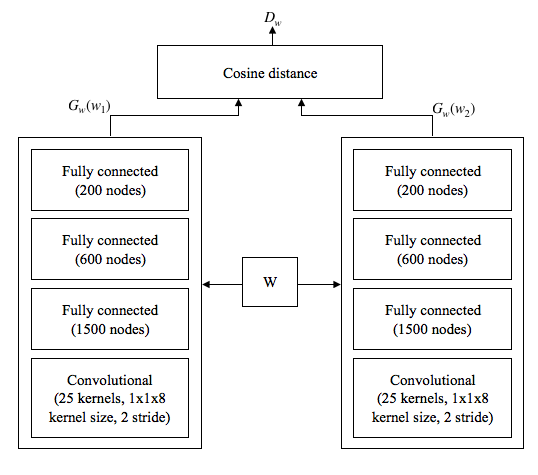Sixth week coding my Google Summer of Code project with Red Hen Lab. I decided to use the time to go into the dialect identification task while the training process of the speech recognition system takes its time. In this post, I’ll briefly go through the data, then I will illustrate the method I am using to build the dialect identification model.

## The data

The MGB-3 data intended for dialect identification includes:

• a total of 73.7 hours of speech, divided into a training set of Al-Jazeera recordings, a small in-domain development set for adaptation and a test set. All the data comes from broadcast news recordings. Each of the utterances in the data belongs to one of the five major Arabic dialects: Egyptian, Levantine, Gulf, North African and Modern Standard Arabic.
• transcriptions generated by an ASR system created for the MGB-2 challenge (2016) are available for the data.
• i-vector features for the speech data and lexical features for the provided text
dsds Training Development Test
Duration 53.6 hours 10 hours 10.1 hours
Table 1: MGB-3 Arabic dialect identification data

## The model

Multiple models were tested by the authors of the MIT-QCRI system .

The main model uses a Siamese neural network. A Siamese neural network is a network that contains two or more sub-networks of identical structure. This network architecture is typically used for comparisons.

As an example example, consider a Siamese neual network with two identical subnetworks. The network accepts two feature vectors at a time, each is input to one of the subnetworks. This leads to the same insight being extracted from both feature vector (remember that the two subnetworks are identical), which results in comparable information.

The authors of the MIT-QCRI system propsed a Siamese neural network with two parallel convolutional neural networks. The input to this network is a pair of i-vectors. The first i-vector is extracted for the utterance whose dialect we wish to identify. The second i-vector is the i-vector representing the dialect itself. This vector is called the i-vector dialect model. To calculate this model, the following equation is used. $\overline{w_d} = \frac{1}{n_d} \sum_{i=1}^{n_d}w_i^d$ Where $n_d$ is the number of utterances in this dialect, and $w_i^d$ is utterance $i$ in dialect $d$. To use the development data for adaptation, the authors used an interpolation approach: $\overline{w_d^{Inter}} = (1 - \gamma)\overline{w_d^{TRN}} + \gamma\overline{w_d^{DEV}}$ where $w_d^{TRN}$ is the training set, $w_d^{DEV}$ is the test set and $\gamma$ is the interpolation parameter. The authors also used a recursive whitening transformation  on i-vectors.Figure 1: Siamese neural network architecture of the MIT-QCRI system

 S. Shon, A. Ali, and J. Glass, “MIT-QCRI Arabic dialect identification system for the 2017 multi-genre broadcast challenge,” in ASRU, 2017.

 Suwon Shon, Seongkyu Mun, and Hanseok Ko, “Recursive Whitening Transformation for Speaker Recognition on Language Mismatched Condition,” in Interspeech, 2017, pp. 2869–2873.